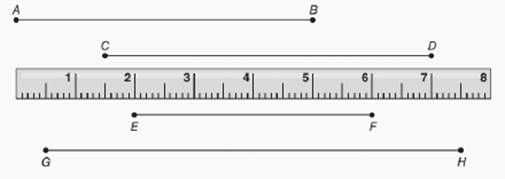Chapter P.CR, Problem 30CRElementary Geometry For College St...

7th Edition
Alexander + 2 others
ISBN: 9781337614085

Solutions

Chapter
SectionElementary Geometry For College St...

7th Edition
Alexander + 2 others
ISBN: 9781337614085
Textbook Problem

For Review Exercises 29 and 30, use the drawing below. Find each indicated length. a) E F b) G HTo determine

a)

To find:

The length of segment EF.

Explanation

Approach:

Measuring line segments:

The instrument used to measure a line segment is a scaled straightedge such as a ruler, a yardstick, or a meter stick.

When we express the length of a line segment, we do not write the bar on top of the name of line segment. Suppose the length of line segment AB- is 10 cm, then we write it as AB=10 cm.

To find the length of line segment using a ruler:

1. Place the ruler so that “0” corresponds to one endpoint of the line segment.

2. Read the length of line segment by reading the number at the remaining endpoint of the line segment.

When “0” is not placed at the endpoint of line segment, the length of line segment is the positive difference between the numbers found at its endpoints

To determine

c)

To find:

The length of segment GH.

Still sussing out bartleby?

Check out a sample textbook solution.

See a sample solution

The Solution to Your Study Problems

Bartleby provides explanations to thousands of textbook problems written by our experts, many with advanced degrees!

Get Started

Find more solutions based on key concepts